# Common Core: High School - Geometry : Congruence

## Example Questions

← Previous 1 3 4 5 6 7 8 9 23 24

### Example Question #1 : Angle, Circle, Perpendicular And Parallel Lines, And Line Segment Definitions: Ccss.Math.Content.Hsg Co.A.1

A truck is traveling down a hill, which of the following statements is/are true?

The truck tires are parallel to the hill.

The body of the truck is parallel to the hill.

The body of the truck is not perpendicular to the hill.

The body of the truck is perpendicular to the hill.

The body of the truck is not perpendicular to the hill.

Explanation:

This question is trying to put a mathematical definition to a real life situation. First, recall the definitions of parallel and perpendicular lines, since those terms are among the answer selections.

Parallel lines: In a plane, parallel lines are lines that will never intersect. This means they have the same slope but different intercepts.

Perpendicular lines: In a plane, perpendicular lines are lines that intersect by creating adegree angle. This also means they have opposite sign, reciprocal slopes.

Now, look at the aspects of this particular problem.

"A truck is traveling down a hill"

From this statement, it cannot be assumed that the hill is a straight line nor can it be assumed that the hill goes on forever. Therefore, the truck and the hill will never be parallel. Also, for these same reasons it is known that the truck will never be perpendicular to the hill. The relation of the truck's tires to the hill will never be parallel since they constantly touch.

Thus, the correct answer choice is,

"The body of the truck is not perpendicular to the hill."

### Example Question #1 : Angle, Circle, Perpendicular And Parallel Lines, And Line Segment Definitions: Ccss.Math.Content.Hsg Co.A.1

A truck is traveling up a hill, which of the following statements is/are true?

The truck tires are parallel to the hill.

The body of the truck is perpendicular to the hill.

The body of the truck is parallel to the hill.

The body of the truck is not perpendicular to the hill.

The body of the truck is not perpendicular to the hill.

Explanation:

This question is trying to put a mathematical definition to a real life situation. First, recall the definitions of parallel and perpendicular lines, since those terms are among the answer selections.

Parallel lines: In a plane, parallel lines are lines that will never intersect. This means they have the same slope but different intercepts.

Perpendicular lines: In a plane, perpendicular lines are lines that intersect by creating adegree angle. This also means they have opposite sign, reciprocal slopes.

Now, look at the aspects of this particular problem.

"A truck is traveling up a hill"

From this statement, it cannot be assumed that the hill is a straight line nor can it be assumed that the hill goes on forever. Therefore, the truck and the hill will never be parallel. Also, for these same reasons it is known that the truck will never be perpendicular to the hill. The relation of the truck's tires to the hill will never be parallel since they constantly touch.

Thus, the correct answer choice is,

"The body of the truck is not perpendicular to the hill."

### Example Question #3 : Congruence

There exists four points on a certain line A. Which of the following is true?

The points are perpendicular

The points are equidistant

The points are collinear

The points are parallel

The points are collinear

Explanation:

First, recall the definitions of the terms in the possible answer choices.

Collinear: Represents points that all fall on the same line.

Equidistance: Represents points that are the same length away from one another.

Parallel: In a plane, parallel lines are lines that will never intersect. This means they have the same slope but different intercepts.

Perpendicular: In a plane, perpendicular lines are lines that intersect by creating adegree angle. This also means they have opposite sign, reciprocal slopes.

Therefore, the correct answer is collinear.

### Example Question #1 : Angle, Circle, Perpendicular And Parallel Lines, And Line Segment Definitions: Ccss.Math.Content.Hsg Co.A.1

The seat of a swing on a swing set is attached to the top horizontal bar by two chains that are exactlyinches from each other and of equal length. The seat of the swing is alsoinches. Which of the following statements describes the geometric relationship between one of the chains and the horizontal bar it is attached to?

Perpendicular

Neither

Parallel

Perpendicular

Explanation:

This question is trying to put a mathematical definition to a real life situation. First, recall the definitions of parallel and perpendicular lines, since those terms are among the answer selections.

Parallel lines: In a plane, parallel lines are lines that will never intersect. This means they have the same slope but different intercepts.

Perpendicular lines: In a plane, perpendicular lines are lines that intersect by creating aangle. This also means they have opposite sign, reciprocal slopes.

Now, look at the aspects of this particular problem.

"The seat of a swing on a swing set is attached to the top horizontal bar by two chains that are exactlyinches from each other and of equal length. The seat of the swing is alsoinches."

The question is asking to define the relationship between one of the chains and the horizontal bar it is attached to. Since the swing will hang directly down from the two chains and the bar is horizontal to ground it can be assumed that the chain and the bar form aangle and thus, they are perpendicular to one another.

### Example Question #1 : High School: Geometry

The seat of a swing on a swing set is attached to the top horizontal bar by two chains that are exactlyinches from each other and of equal length. The seat of the swing is alsoinches. Which of the following statements describes the geometric relationship between the two chains?

Perpendicular

Neither

Parallel

Parallel

Explanation:

This question is trying to put a mathematical definition to a real life situation. First, recall the definitions of parallel and perpendicular lines, since those terms are among the answer selections.

Parallel lines: In a plane, parallel lines are lines that will never intersect. This means they have the same slope but different intercepts.

Perpendicular lines: In a plane, perpendicular lines are lines that intersect by creating aangle. This also means they have opposite sign, reciprocal slopes.

Now, look at the aspects of this particular problem.

"The seat of a swing on a swing set is attached to the top horizontal bar by two chains that are exactlyinches from each other and of equal length. The seat of the swing is alsoinches."

The question is asking to define the relationship between the two chains that hold the swing to the swing set. Since the two chains are exactlyinches apart from one another and attached to the pole which is horizontal from the swing and the swing seat itself isinches, it is concluded that the two chains are parallel to one another.

### Example Question #2 : High School: Geometry

The seat of a swing on a swing set is attached to the top horizontal bar by two chains that are exactlyinches from each other and of equal length. The seat of the swing is alsoinches. Which of the following statements describes the geometric relationship between the horizontal bar and the swing?

Perpendicular

Parallel

Neither

Parallel

Explanation:

This question is trying to put a mathematical definition to a real life situation. First, recall the definitions of parallel and perpendicular lines, since those terms are among the answer selections.

Parallel lines: In a plane, parallel lines are lines that will never intersect. This means they have the same slope but different intercepts.

Perpendicular lines: In a plane, perpendicular lines are lines that intersect by creating aangle. This also means they have opposite sign, reciprocal slopes.

Now, look at the aspects of this particular problem.

"The seat of a swing on a swing set is attached to the top horizontal bar by two chains that are exactlyinches from each other and of equal length. The seat of the swing is alsoinches."

The question is asking to define the relationship between the horizontal bar and the swing seat. Since the two chains are exactlyinches apart from one another and of equal length and attached to the pole which is horizontal from the swing and the swing seat itself isinches, it is concluded that the seat and the horizontal bar are parallel to one another.

### Example Question #2 : Angle, Circle, Perpendicular And Parallel Lines, And Line Segment Definitions: Ccss.Math.Content.Hsg Co.A.1

A circular pizza is cut intoequal slices. Which of the following is an accurate mathematical description of one of the pizza slices?

The central angle of one pizza slice isdegrees.

The central angle of one pizza slice isdegrees.

The central angle of one pizza slice isdegrees.

The central angle of one pizza slice isdegrees.

The central angle of one pizza slice isdegrees.

Explanation:

This question is trying to put a mathematical definition to a real life situation. First, recall the definitions of circles and corresponding angles.

A central angle is known as the angle of a circle where the vertex of the angle is located at the center of the circle.

A circle is composed of 360 degrees.

Knowing these characteristics, solve for the central angle of one slice of pizza.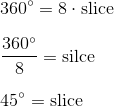"The central angle of one pizza slice isdegrees."

### Example Question #1 : Angle, Circle, Perpendicular And Parallel Lines, And Line Segment Definitions: Ccss.Math.Content.Hsg Co.A.1

A circular pizza that has a radius ofinches and is cut intoequal slices. Which of the following is an accurate mathematical description of one of the pizza slices?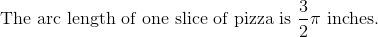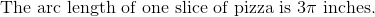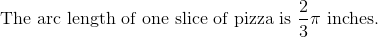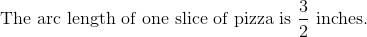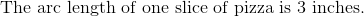Explanation:

This question is trying to put a mathematical definition to a real life situation. First, recall the definitions of circles and corresponding angles.

A central angle is known as the angle of a circle where the vertex of the angle is located at the center of the circle.

A circle is composed of 360 degrees.

The circumference of a circle is the length around the circle and the radius is the length from the center of the circle to any point on the circle's edge.

For this particular question, calculate the circumference and then calculate the arc length of each slice pizza slice.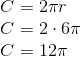Since there are 8 equal slices, divide the circumference by 8.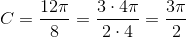"The arc length of one slice of pizza is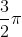inches."

### Example Question #1 : Angle, Circle, Perpendicular And Parallel Lines, And Line Segment Definitions: Ccss.Math.Content.Hsg Co.A.1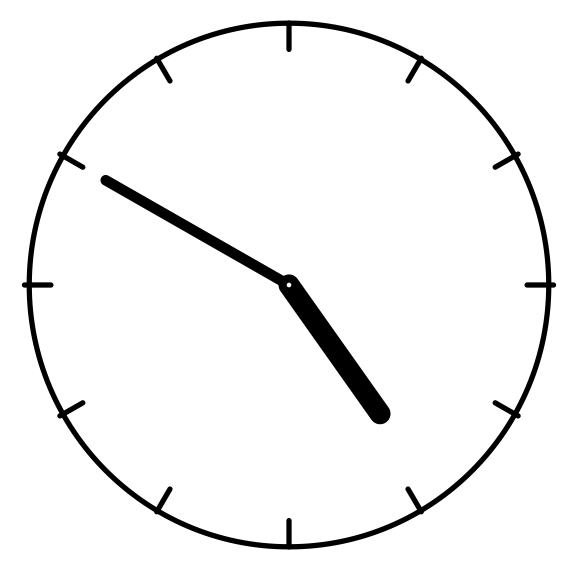Looking at the given clock where the radius isinches, which of the following statements accurately describes the space between the hour and minute hand?

The area between the hour and minute hand is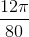The area between the hour and minute hand is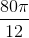The area between the hour and minute hand is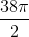The area between the hour and minute hand is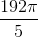The area between the hour and minute hand isExplanation:

This question is trying to put a mathematical definition to a real life situation. First, recall the definitions of circles and corresponding angles.

A central angle is known as the angle of a circle where the vertex of the angle is located at the center of the circle.

A circle is composed of 360 degrees.

The area of a circle is found by using the formula.For this particular problem first calculate the area of the clock.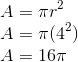Now, since the clock reads 4:50, the distance between the hour and minute hands is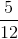of the total clock. From here, calculate the area between the two hands.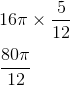The area between the hour and minute hand is.

### Example Question #1 : Angle, Circle, Perpendicular And Parallel Lines, And Line Segment Definitions: Ccss.Math.Content.Hsg Co.A.1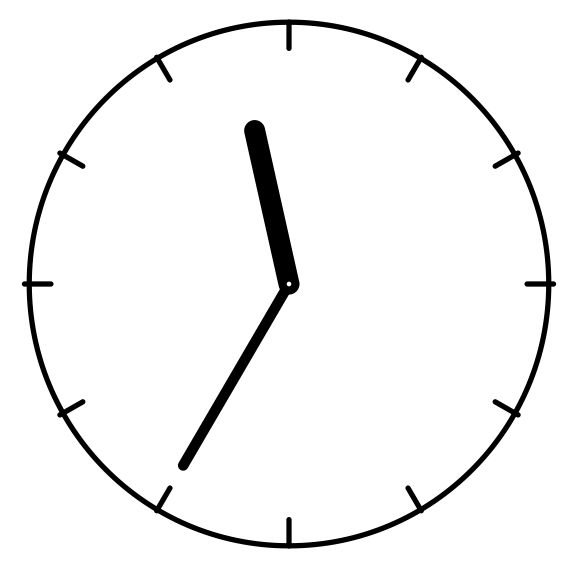Looking at the given clock where the radius isinches, which of the following statements accurately describes the space between the hour and minute hand (Going clockwise)?

The angle between the hour and minute hand is greater thandegrees.

The angle between the hour and minute hand is less thandegrees.

The angle between the hour and minute hand is less thandegrees.

The angle between the hour and minute hand is greater thandegrees.

The angle between the hour and minute hand is greater thandegrees.

Explanation:

This question is trying to put a mathematical definition to a real life situation. First, recall the definitions of circles and corresponding angles.

A central angle is known as the angle of a circle where the vertex of the angle is located at the center of the circle.

A circle is composed of 360 degrees.

Also recall that a straight line measures 180 degrees.

Looking at the given clock, it is seen that a straight line can be created by connecting the 12 and 6 on the clock. Since the clock reads 11:35 the angle between the hour and minute hand is greater than 180 degrees because the hour hand is behind the 12 and the minute hand is behind the 6 on the clock.← Previous 1 3 4 5 6 7 8 9 23 24

### All Common Core: High School - Geometry Resources